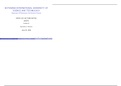• Followers
• Following

### 36 items#### Limits at infinity and Tangent lines

(0)
\$3.49
0x  sold

Limits at infinity are used to describe the behavior of functions as the independent variable increases or decreases without bound. If a function approaches a numerical value L in either of these situations, write. and f( x) is said to have a horizontal asymptote at y = L.) Find the first derivative of f(x). 2) Plug x value of the indicated point into f '(x) to find the slope at x. 3) Plug x value into f(x) to find the y coordinate of the tangent point. 4) Combine the slope from step 2 and point...

i x
•  Book
• Class notes
•  • 11 pages •